# Python的txt文本操作-读、写

## 读取txt文本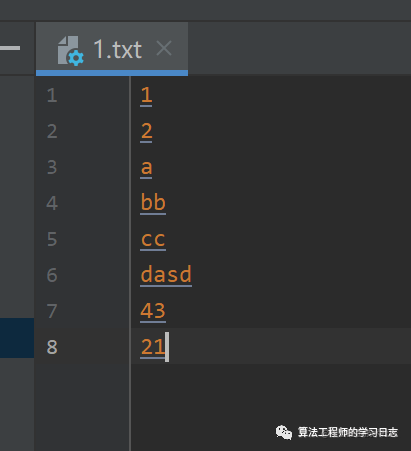``````with open("1.txt", "r") as f:  # 打开文件    data = f.read()  # 读取文件    print(data)    print(type(data))    for i in data:        print(i)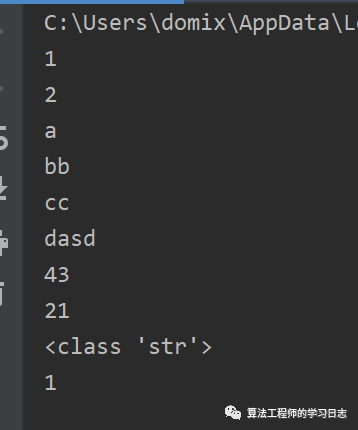``````with open("1.txt", "r") as f:    data = f.readline()    print(data)    print(type(data))    for i in data:        print(i)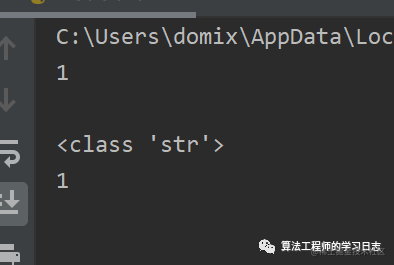``````with open("1.txt", "r") as f:    data = f.readlines()    print(data)    print(type(data))    for i in data:        print(i)``````with open("1.txt", "r") as f:    data = f.readlines()    print(data)    print(type(data))    for i in data:        print(i.strip())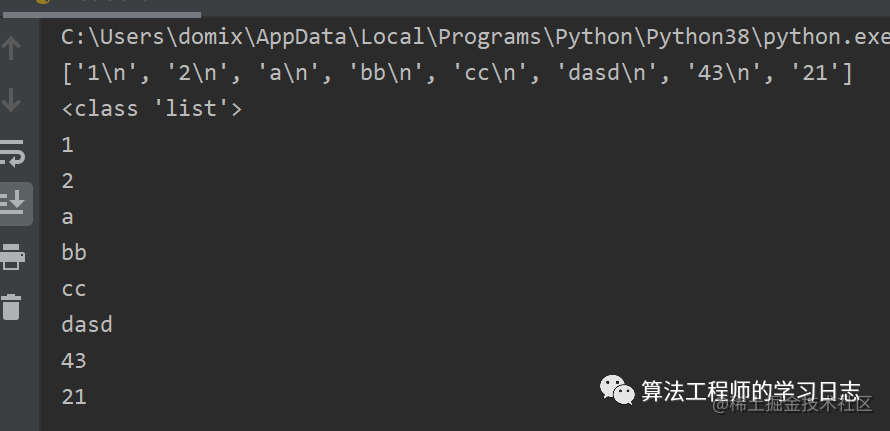## 写入txt文本​​​​​​​

``````with open("2.txt", "w") as f:    f.write("这是个测试！")      # 自带文件关闭功能，不需要再写f.close()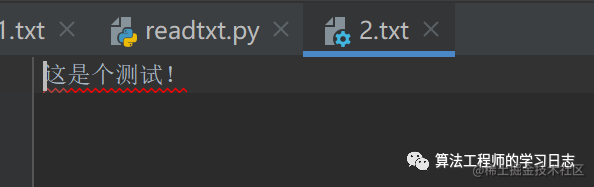## 读写模式

• r :   读取文件，若文件不存在则会报错
• w:   写入文件，若文件不存在则会先创建再写入，会覆盖原文件
• a :   写入文件，若文件不存在则会先创建再写入，但不会覆盖原文件，而是追加在文件末尾
• rb,wb：  分别于r,w类似，但是用于读写二进制文件
• r+ :   可读、可写，文件不存在也会报错，写操作时会覆盖
• w+ :   可读，可写，文件不存在先创建，会覆盖
• a+ :  可读、可写，文件不存在先创建，不会覆盖，追加在末尾